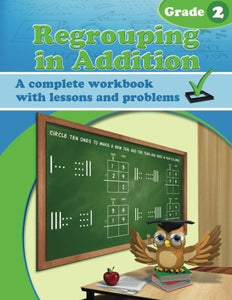# Regrouping In Addition Workbook

• Publish Date: 2016-01-01
• Binding: Paperback
• Author: Maria Miller
• \$17.97
• Save \$13.94

Attention: For textbook, access codes and supplements are not guaranteed with used items.

Regrouping in Addition Workbook is ideal for second grade. It deals with addition within 0-100, both mentally and in columns, especially concentrating on regrouping in addition (carrying).

Mental math

Mental math is important because it builds number sense. We study adding mentally a two-digit number and a single-digit number where the answer goes to the next ten (problems such as 36 + 8 or 45 + 9). These additions use the helping problem composed of the single-digit numbers (6 + 8 or 5 + 9). The child knows that 6 + 8 fills the first ten and is four more than the ten. He/she will learn to use that fact when adding 36 + 8. The sum 36 + 8 fills the next whole ten (40), and is four more than that, or 44.

Regrouping in tens

We also study adding two-digit numbers in columns, and regrouping with tens, or carrying, which is illustrated and explained in detail with the help of visual models. These visual models take the place of base-ten blocks or other manipulatives. You are welcome to use actual manipulatives if you prefer. The main concept to understand is that 10 ones make a new ten, and this new ten is regrouped with the other tens, written using a little 1 in the tens column.

In order to prepare for adding three or four two-digit numbers in columns, we practice explicitly how to add 3 or 4 single-digit numbers, such as 7 + 8 + 6 + 4, and the principle of adding in parts (such as 13 + 16 is the same as 10 + 10 and 3 + 6).

The lessons also include lots of word problems, review of even and odd numbers, and occasional review problems about doubling.

Later on, in the lesson Adding 3-Digit Numbers in Columns, the student adds three-digit numbers, regrouping in tens, but there is no regrouping in hundreds. Students already know how to regroup two-digit numbers, so this lesson only extends that knowledge to numbers that have three digits.

In the next lesson, students regroup ten tens as a hundred (or carry to the hundreds). This is first illustrated with visual models. You can do the exercises that include visual models with manipulatives instead (base ten blocks), if you prefer.

Then we study regrouping twice: 10 ones form a new ten, and then 10 tens form a new hundred. Again, students work first with visual models, with the aim of helping them to understand the concept itself. Then, they do the process with numbers only, adding in columns.